Circle Product Theorems

Students will use dynamic models to find patterns. These patterns are the Chord-Chord, Secant-Secant, and Secant-Tangent Theorems.
•TI-84 Plus CE
•TI-84 Plus C Silver Edition
• TI-84 Plus Silver Edition
• TI-84 Plus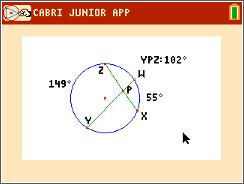Angles and Arcs

Students explore angles constructed in a circle and how their measures are related to the measures of the intercepted arcs.
•TI-84 Plus CE
•TI-84 Plus C Silver Edition
• TI-84 Plus Silver Edition
• TI-84 Plus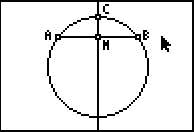Chords and Circles

Students explore how the chord in a circle is related to its perpendicular bisector.
•TI-84 Plus CE
•TI-84 Plus C Silver Edition
• TI-84 Plus Silver Edition
• TI-84 Plus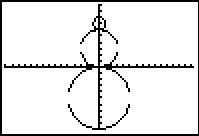Exploring Circle Equations

Students explore the equation of a circle by connecting the coordinates of the center of the circle and the length of the radius to the corresponding parts of the equation.
•TI-84 Plus CE
•TI-84 Plus C Silver Edition
• TI-84 Plus Silver Edition
• TI-84 Plus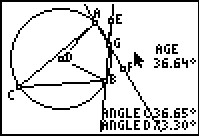Inscribed Angles Theorem

Students investigate the relationship between inscribed angles and central angles, the Inscribed Angle Theorem.
•TI-84 Plus CE
•TI-84 Plus C Silver Edition
• TI-84 Plus Silver Edition
• TI-84 Plus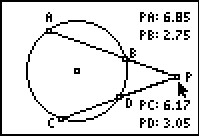••